# Thermodynamic, part 1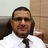- Assist.Prof of Laser Physics & Photonics um Ibb University, Yemen + Jazan University, KSA
2. Sep 2016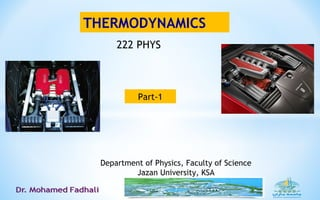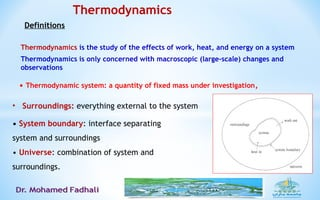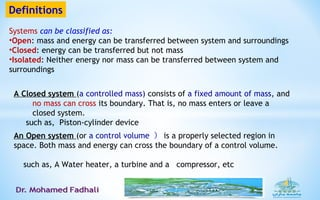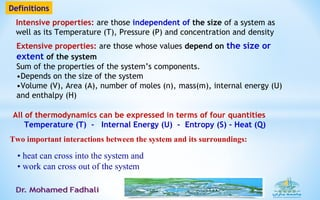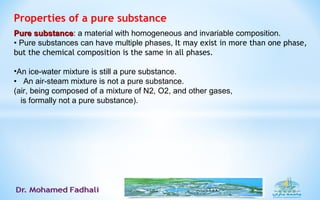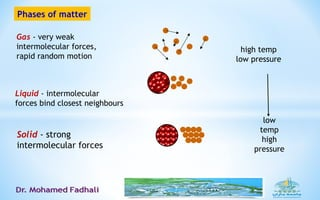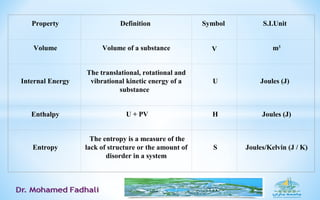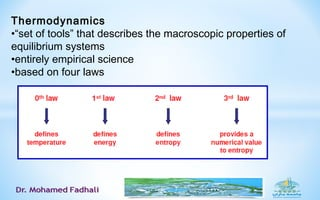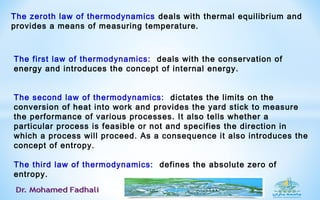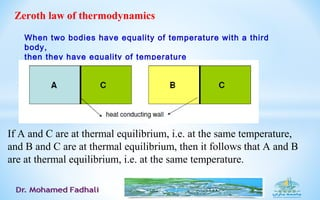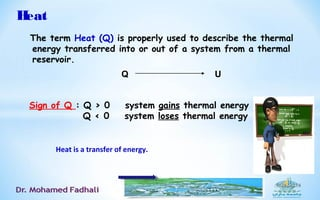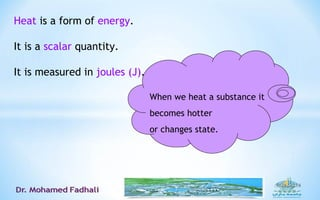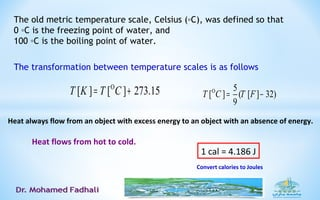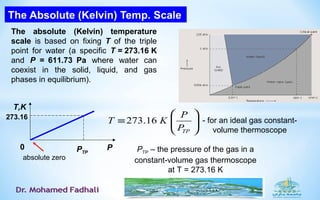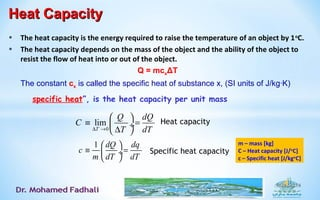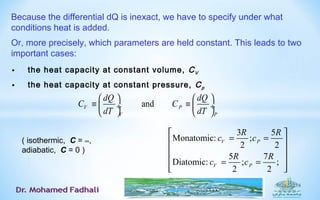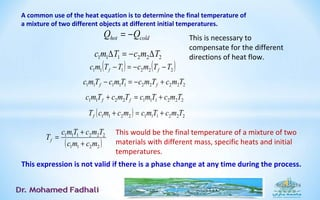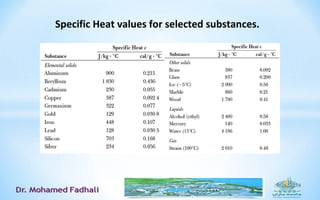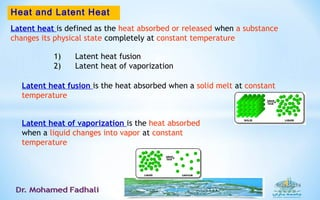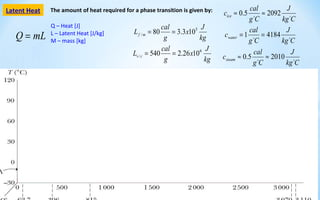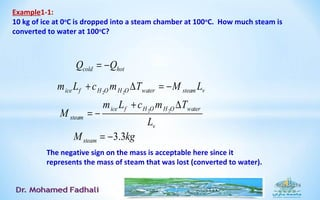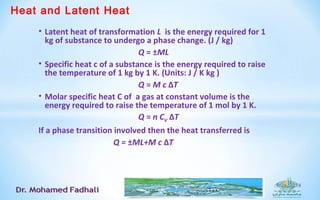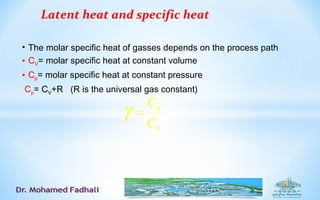1 von 24

### Thermodynamic, part 1

• 1. THERMODYNAMICS Department of Physics, Faculty of Science Jazan University, KSA Part-1 222 PHYS
• 2. Thermodynamics is the study of the effects of work, heat, and energy on a system Thermodynamics is only concerned with macroscopic (large-scale) changes and observations Thermodynamics • Thermodynamic system: a quantity of fixed mass under investigation, Definitions • System boundary: interface separating system and surroundings • Universe: combination of system and surroundings. • Surroundings: everything external to the system
• 3. Definitions Systems can be classified as: •Open: mass and energy can be transferred between system and surroundings •Closed: energy can be transferred but not mass •Isolated: Neither energy nor mass can be transferred between system and surroundings A Closed system (a controlled mass) consists of a fixed amount of mass, and no mass can cross its boundary. That is, no mass enters or leave a closed system. 　 such as, Piston-cylinder device An Open system (or a control volume ） is a properly selected region in space. Both mass and energy can cross the boundary of a control volume. such as, A Water heater, a turbine and a compressor, etc
• 4. Definitions Intensive properties: are those independent of the size of a system as well as its Temperature (T), Pressure (P) and concentration and density Extensive properties: are those whose values depend on the size or extent of the system Sum of the properties of the system’s components. •Depends on the size of the system •Volume (V), Area (A), number of moles (n), mass(m), internal energy (U) and enthalpy (H) Two important interactions between the system and its surroundings: • heat can cross into the system and • work can cross out of the system All of thermodynamics can be expressed in terms of four quantities Temperature (T) - Internal Energy (U) - Entropy (S) - Heat (Q)
• 5. Thermodynamics provides a framework of relating the macroscopic properties of a system to one another. In general: Change where system is always in thermal equilibrium: { reversible process} Change where system is not always in thermal equilibrium: {irreversible process} Examples of irreversible processes: • Free expansion - melting of ice in warmer liquid - frictional heating Types of the questions that Thermodynamics addresses: • How does an engine work? What is its maximum efficiency? • How does a refrigerator work? What is its maximum efficiency? • How much energy do we need to add to a kettle of water to change it to steam?
• 6. Properties of a pure substance Pure substancePure substance: a material with homogeneous and invariable composition. • Pure substances can have multiple phases, It may exist in more than one phase, but the chemical composition is the same in all phases. •An ice-water mixture is still a pure substance. • An air-steam mixture is not a pure substance. (air, being composed of a mixture of N2, O2, and other gases, is formally not a pure substance).
• 7. Phases of matter Gas - very weak intermolecular forces, rapid random motion Liquid - intermolecular forces bind closest neighbours Solid - strong intermolecular forces low temp high pressure high temp low pressure
• 8.  Property Definition  Symbol  S.I.Unit   Volume Volume of a substance V  m3  Internal Energy The translational, rotational and  vibrational kinetic energy of a  substance    U Joules (J)   Enthalpy  U + PV  H  Joules (J)  Entropy  The entropy is a measure of the  lack of structure or the amount of  disorder in a system  S  Joules/Kelvin (J / K)
• 9. Thermodynamics •“set of tools” that describes the macroscopic properties of equilibrium systems •entirely empirical science •based on four laws
• 10. The zeroth law of thermodynamics deals with thermal equilibrium and provides a means of measuring temperature. The first law of thermodynamics: deals with the conservation of energy and introduces the concept of internal energy.     The second law of thermodynamics: dictates the limits on the conversion of heat into work and provides the yard stick to measure the performance of various processes. It also tells whether a particular process is feasible or not and specifies the direction in which a process will proceed. As a consequence it also introduces the concept of entropy.   The third law of thermodynamics: defines the absolute zero of entropy.
• 11. Zeroth law of thermodynamics When two bodies have equality of temperature with a third body, then they have equality of temperature If A and C are at thermal equilibrium, i.e. at the same temperature,  and B and C are at thermal equilibrium, then it follows that A and B  are at thermal equilibrium, i.e. at the same temperature.
• 12. Heat Sign of Q : Q > 0 system gains thermal energy Q < 0 system loses thermal energy The term Heat (Q) is properly used to describe the thermal energy transferred into or out of a system from a thermal reservoir. Q U Heat is a transfer of energy.
• 13. Heat is a form of energy. It is a scalar quantity. It is measured in joules (J). When we heat a substance it becomes hotter or changes state.
• 14. The old metric temperature scale, Celsius (◦C), was defined so that 0 ◦C is the freezing point of water, and 100 ◦C is the boiling point of water. The transformation between temperature scales is as follows Heat always flow from an object with excess energy to an object with an absence of energy. Heat flows from hot to cold. 1 cal = 4.186 J Convert calories to Joules O [ ] [ ] 273.15T K T C= + O 5 [ ] ( [ ] 32) 9 T C T F= −
• 15. The Absolute (Kelvin) Temp. Scale The absolute (Kelvin) temperature scale is based on fixing T of the triple point for water (a specific T = 273.16 K and P = 611.73 Pa where water can coexist in the solid, liquid, and gas phases in equilibrium).       ≡ TPP P KT 16.273 - for an ideal gas constant- volume thermoscope absolute zero T,K PPTP 273.16 PTP – the pressure of the gas in a constant-volume gas thermoscope at T = 273.16 K 0
• 16. Heat CapacityHeat Capacity • The heat capacity is the energy required to raise the temperature of an object by 1o C. • The heat capacity depends on the mass of the object and the ability of the object to resist the flow of heat into or out of the object. Q = mcxΔT • The constant cx is called the specific heat of substance x, (SI units of J/kg·K) 1 dQ dq c m dT dT   ≡ = ÷   Specific heat capacity specific heat”, is the heat capacity per unit mass m – mass [kg] C – Heat capacity [J/o C] c – Specific heat [J/kgo C] 0 lim T Q dQ C T dT∆ →   ≡ = ÷ ∆  Heat capacity
• 17. Because the differential dQ is inexact, we have to specify under what conditions heat is added. Or, more precisely, which parameters are held constant. This leads to two important cases: • the heat capacity at constant volume, CV • the heat capacity at constant pressure, Cp andV P V P dQ dQ C C dT dT     ≡ ≡ ÷  ÷     ( isothermic, C = ∞, adiabatic, C = 0 ) 3 5 Monatomic: ; 2 2 5 7 Diatomic: ; ; 2 2 V P V P R R c c R R c c   = =     = =   
• 18. A common use of the heat equation is to determine the final temperature of a mixture of two different objects at different initial temperatures. coldhot QQ −= 222111 TmcTmc ∆−=∆ This is necessary to compensate for the different directions of heat flow. ( ) ( )222111 TTmcTTmc ff −−=− 2222211111 TmcTmcTmcTmc ff +−=− 2221112211 TmcTmcTmcTmc ff +=+ ( ) 2221112211 TmcTmcmcmcTf +=+ ( )2211 222111 mcmc TmcTmc Tf + + = This would be the final temperature of a mixture of two materials with different mass, specific heats and initial temperatures. This expression is not valid if there is a phase change at any time during the process.
• 19. Specific Heat values for selected substances.
• 20. Heat and Latent Heat Latent heat is defined as the heat absorbed or released when a substance changes its physical state completely at constant temperature 1) Latent heat fusion 2) Latent heat of vaporization Latent heat fusion is the heat absorbed when a solid melt at constant temperature Latent heat of vaporization is the heat absorbed when a liquid changes into vapor at constant temperature
• 21. Latent Heat The amount of heat required for a phase transition is given by: mLQ = Q – Heat [J] L – Latent Heat [J/kg] M – mass [kg] Ckg J Cg cal cwater  41841 == Ckg J Cg cal cice  20925.0 ≈≈ Ckg J Cg cal csteam  20105.0 ≈≈ kg J x g cal L mf 5 / 103.380 == kg J x g cal L cv 6 / 1026.2540 ==
• 22. Example1-1: 10 kg of ice at 0o C is dropped into a steam chamber at 100o C. How much steam is converted to water at 100o C? hotcold QQ −= 2 2ice f H O H O water steam vm L c m T M L+ ∆ = − 2 2ice f H O H O water steam v m L c m T M L + ∆ = − kgMsteam 3.3−= The negative sign on the mass is acceptable here since it represents the mass of steam that was lost (converted to water).
• 23. Heat and Latent Heat • Latent heat of transformation L is the energy required for 1 kg of substance to undergo a phase change. (J / kg) Q = ±ML • Specific heat c of a substance is the energy required to raise the temperature of 1 kg by 1 K. (Units: J / K kg ) Q = M c ΔT • Molar specific heat C of a gas at constant volume is the energy required to raise the temperature of 1 mol by 1 K. Q = n CV ΔT If a phase transition involved then the heat transferred is Q = ±ML+M c ΔT
• 24. Latent heat and specific heat • The molar specific heat of gasses depends on the process path • CV= molar specific heat at constant volume • Cp= molar specific heat at constant pressure Cp= CV+R (R is the universal gas constant) VC Cp =γ